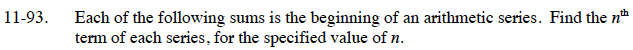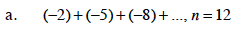Home > CCA2 > Chapter 11 > Lesson 11.3.1 > Problem11-93

11-93.
1. Each of the following sums is the beginning of an arithmetic series. Find the nth term of each series, for the specified value of n. Homework Help ✎

1. (−2) + (−5) + (−8) + ..., n = 12

2. 9 + 15 + 21 + ..., n = 20How is this sequence progressing?
How can you use that to determine what the 12th term will be?

−35Use the same method as part (a).